Download the eSaral app and start learning from Kota's top IITians and doctors.

# NCERT Solutions for Class 11 Physics chapter 8 - Gravitation - Free PDF DownloadHey, are you a class 11 student and looking for ways to download NCERT Solutions for Class 11 Physics chapter 8 "Gravitation"? If yes. Then read this post till the end.

In this article, we have listed NCERT solution for class 11 Physics Chapter 8 in PDF that are prepared by Kota’s top IITian’s Faculties by keeping Simplicity in mind.

If you want to learn and understand class 11 Physics chapter 8 "Gravitation" in an easy way then you can use these solutions PDF.

NCERT Solutions helps students to Practice important concepts of subjects easily. Class 11 Physics solutions provide detailed explanations of all the NCERT questions that students can use to clear their doubts instantly.

If you want to score high in your class 11 Physics Exam then it is very important for you to have a good knowledge of all the important topics, so to learn and practice those topics you can use eSaral NCERT Solutions.

So, without wasting more time Let’s start.

### Download The PDF of NCERT Solutions for Class 11 Physics Chapter 8 "Gravitation"

Question 1:
(a) You can shield a charge from electrical forces by putting it inside a hollow conductor. Can you shield a body from the gravitational influence of nearby matter by putting it inside a hollow sphere or by some other means?
(b) An astronaut inside a small space ship orbiting around the earth cannot detect gravity. If the space station orbiting around the earth has a large size, can he hope to detect gravity?
(c) If you compare the gravitational force on the earth due to the sun to that due to the moon, you would find that the Sun’s pull is greater than the moon’s pull. (You can check this yourself using the data available in the succeeding exercises). However, the tidal effect of the moon’s pull is greater than the tidal effect of sun. Why?

Solution.
(a) Gravitational influence of matter on nearby objects cannot be screened by any means. This is because gravitational force unlike electrical forces is independent of the nature of the material medium. Also, it is independent of the status of other objects.
(b) If the size of the space station is large enough, then the astronaut will detect the change in Earth’s gravity (g).
(c) Tidal effect depends inversely upon the cube of the distance while, gravitational force depends inversely on the square of the distance. Since the distance between the Moon and the Earth is smaller than the distance between the Sun and the Earth, the tidal effect of the Moon’s pull is greater than the tidal effect of the Sun’s pull.

Question 2:
Choose the correct alternative:
(a) Acceleration due to gravity increases/decreases with increasing altitude
(b) Acceleration due to gravity increases/decreases with increasing depth. (assume the earth to be a sphere of uniform density)
(c) Acceleration due to gravity is independent of mass of the earth/mass of the body.
(d) The formula $-G M m\left(1 / r_{2}-1 / r_{1}\right)$ is more/less accurate than the formula $m g\left(r_{2}-r_{1}\right)$ for the difference of potential energy between two points $r_{2}$ and $r_{1}$ distance away from the centre of the earth

Solution.
(a) Decreases
(b) Decreases
(c) Mass of the body
(d) More
Explanation:
(a) Acceleration due to gravity at depth $h$ is given by the relation:
$\mathrm{g}_{h}=\left(1-\frac{2 h}{R_{\mathrm{e}}}\right) \mathrm{g}$
Where,
$R_{e}=$ Radius of the Earth
$\mathrm{g}=$ Acceleration due to gravity on the surface of the Earth
It is clear from the given relation that acceleration due to gravity decreases with an increase in height.
(b) Acceleration due to gravity at depth $d$ is given by the relation:
$d=\left(1-\frac{d}{R_{\mathrm{e}}}\right) \mathrm{g}$
It is clear from the given relation that acceleration due to gravity decreases with an increase in depth.
(c) Acceleration due to gravity of body of mass $m$ is given by the relation:
$\mathrm{g}=\frac{\mathrm{G} M}{R^{2}}$
Where,
$\mathrm{G}=$ Universal gravitational constant
$M=$ Mass of the Earth
$R=$ Radius of the Earth
Hence, it can be inferred that acceleration due to gravity is independent of the mass of the body.
(d) Gravitational potential energy of two points $r_{2}$ and $r_{1}$ distance away from the centre of the Earth is respectively given by:
$V\left(r_{1}\right)=-\frac{\mathrm{G} m M}{r_{1}}$
$V\left(r_{2}\right)=-\frac{\mathrm{G} m M}{r_{2}}$
\begin{aligned}
&\therefore \text { Difference in potential energy, } V=V\left(r_{2}\right)-V\left(r_{1}\right)=-\mathrm{G} m M\left(\frac{1}{r_{2}}-\frac{1}{r_{1}}\right)\\
&\text { Hence, this formula is more accurate than the formula } m g\left(r_{2}-r_{1}\right) \text { . }
\end{aligned}

Question 3:
Suppose there existed a planet that went around the sun twice as fast as the earth.What would be its orbital size as compared to that of the earth?

Solution.
Answer: Lesser by a factor of $0.63$
Time taken by the Earth to complete one revolution around the Sun,
$T_{\mathrm{e}}=1$ year
Orbital radius of the Earth in its orbit, $R_{\mathrm{e}}=1 \mathrm{AU}$
Time taken by the planet to complete one revolution around the Sun, $T_{p}=\frac{1}{2} T_{e}=\frac{1}{2}$ year
Orbital radius of the planet $=R_{\mathrm{p}}$
From Kepler's third law of planetary motion, we can write:
$\left(\frac{R_{p}}{R_{e}}\right)^{3}=\left(\frac{T_{P}}{T_{e}}\right)^{2}$
$\frac{R_{p}}{R_{e}}=\left(\frac{T_{P}}{T_{e}}\right)^{\frac{2}{3}}$
$=\left(\frac{\frac{1}{2}}{1}\right)^{\frac{2}{3}}=(0.5)^{\frac{2}{3}}=0.63$
Hence, the orbital radius of the planet will be 0.63 times smaller than that of the Earth.

Question 4:
lo, one of the satellites of Jupiter, has an orbital period of $1.769$ days and the radius of the orbit is $4.22 \times 10^{8} \mathrm{~m}$. Show that the mass of Jupiter is about one-thousandth that of the sun.

Solution.
Orbital period of $I_{0}, T_{l o}=1.769$ days $=1.769 \times 24 \times 60 \times 60 \mathrm{~s}$
Orbital radius of $I_{0}, R_{I o}=4.22 \times 10^{8} \mathrm{~m}$
Satellite $I_{0}$ is revolving around the Jupiter
Mass of the latter is given by the relation:
M_{J}=\frac{4 \pi^{2} R_{\pm}^{3}}{\mathrm{G} T_{l o}^{2}}\ldots (i) Where, M_{J}= Mass of Jupiter \mathrm{G}= Universal gravitational constant Orbital period of the Earth, T_{e}=365.25 days =365.25 \times 24 \times 60 \times 60 \mathrm{~s} Orbital radius of the Earth, R_{e}=1 \mathrm{AU}=1.496 \times 10^{11} \mathrm{~m} Mass of Sun is given as: M_{\mathrm{s}}=\frac{4 \pi^{2} R_{c}^{3}}{\mathrm{G} T_{e}^{2}}... (ii) \therefore \frac{M_{s}}{M_{\nu}}=\frac{4 \pi^{2} R_{e}^{3}}{\mathrm{G} T_{e}^{2}} \times \frac{\mathrm{G} T_{b o}^{2}}{4 \pi^{2} R_{b o}^{3}}=\frac{R_{e}^{3}}{R_{b o}^{3}} \times \frac{T_{b o}^{2}}{T_{e}^{2}} =\left(\frac{1.769 \times 24 \times 60 \times 60}{365.25 \times 24 \times 60 \times 60}\right)^{2} \times\left(\frac{1.496 \times 10^{11}}{4.22 \times 10^{8}}\right)^{3} =1045.04 \therefore \frac{M_{S}}{M_{J}} \sim 1000 M_{S} \sim 1000 \times M Hence, it can be inferred that the mass of Jupiter is about one-thousandth that of the Sun. Question 5: revolution? Take the diameter of the Milky Way to be 10^{5} \mathrm{ly} Solution. Mass of our galaxy Milky Way, M=2.5 \times 10^{11} solar mass Solar mass = Mass of Sun =2.0 \times 10^{36} \mathrm{~kg} Mass of our galaxy, M=2.5 \times 10^{11} \times 2 \times 10^{36}=5 \times 10^{41} \mathrm{~kg} Diameter of Milky Way, d=10^{5} \mathrm{ly} Radius of Milky Way, r=5 \times 10^{4} \mathrm{ly} 1 \mathrm{ly}=9.46 \times 10^{15} \mathrm{~m} \therefore r=5 \times 10^{4} \times 9.46 \times 10^{15} =4.73 \times 10^{20} \mathrm{~m} Since a star revolves around the galactic centre of the Milky Way, its time period is given by the relation: T=\left(\frac{4 \pi^{2} r^{3}}{\mathrm{G} M}\right)^{\frac{1}{2}} =\left(\frac{4 \times(3.14)^{2} \times(4.73)^{3} \times 10^{60}}{6.67 \times 10^{-11} \times 5 \times 10^{41}}\right)^{\frac{1}{2}}=\left(\frac{39.48 \times 105.82 \times 10^{30}}{33.35}\right)^{\frac{1}{2}} =\left(125.27 \times 10^{30}\right)^{\frac{1}{2}}=1.12 \times 10^{16} \mathrm{~s} l year =365 \times 324 \times 60 \times 60 \mathrm{~s} 1 \mathrm{~s}=\frac{1}{365 \times 24 \times 60 \times 60} years \begin{aligned} \therefore 1.12 \times 10^{16} \mathrm{~s} &=\frac{1.12 \times 10^{16}}{365 \times 24 \times 60 \times 60} \\ &=3.55 \times 10^{8} \text { years } \end{aligned} Question 6: Choose the correct alternative: (a) If the zero of potential energy is at infinity, the total energy of an orbiting satellite is negative of its kinetic/potential energy. (b) The energy required to launch an orbiting satellite out of earth’s gravitational influence is more/less than the energy required to project a stationary object at the same height (as the satellite) out of earth’s influence. Solution. (a) Kinetic energy (b) Less (a) Total mechanical energy of a satellite is the sum of its kinetic energy (always positive) and potential energy (may be negative). At infinity, the gravitational potential energy of the satellite is zero. As the Earth-satellite system is a bound system, the total energy of the satellite is negative. Thus, the total energy of an orbiting satellite at infinity is equal to the negative of its kinetic energy. (b) An orbiting satellite acquires a certain amount of energy that enables it to revolve around the Earth. This energy is provided by its orbit. It requires relatively lesser energy to move out of the influence of the Earth’s gravitational field than a stationary object on the Earth’s surface that initially contains no energy. Question 7: Does the escape speed of a body from the earth depend on (a) the mass of the body, (b) the location from where it is projected, (c) the direction of projection, (d) the height of the location from where the body is launched? Solution. (a) No (b) No (c) No (d) Yes Escape velocity of a body from the Earth is given by the relation: v_{\mathrm{esc}}=\sqrt{2 \mathrm{~g} R}\ldots (i)
$\mathrm{g}=$ Acceleration due to gravity
$R=$ Radius of the Earth
It is clear from equation (i) that escape velocity vesc is independent of the mass of the body and the direction of its projection. However, it depends on gravitational potential at the point from where the body is launched. Since this potential marginally depends on the height of the point, escape velocity also marginally depends on these factors.

Question 8:
A comet orbits the Sun in a highly elliptical orbit. Does the comet have a constant (a) linear speed, (b) angular speed, (c) angular momentum, (d) kinetic energy, (e) potential energy, (f) total energy throughout its orbit? Neglect any mass loss of the comet when it comes very close to the Sun.

Solution.
(a) No
(b) No
(c) Yes
(d) No
(e) No
(f) Yes
Angular momentum and total energy at all points of the orbit of a comet moving in a highly elliptical orbit around the Sun are constant. Its linear speed, angular speed, kinetic, and potential energy varies from point to point in the orbit.

Question 9:
Which of the following symptoms is likely to afflict an astronaut in space (a) swollen feet, (b) swollen face, (c) headache, (d) orientational problem?

Solution.
(b), (c), and (d)
(a) Legs hold the entire mass of a body in standing position due to gravitational pull. In space, an astronaut feels weightlessness because of the absence of gravity. Therefore, swollen feet of an astronaut do not affect him/her in space.
(b) A swollen face is caused generally because of apparent weightlessness in space. Sense organs such as eyes, ears nose, and mouth constitute a person's face. This symptom can affect an astronaut in space.
(c) Headaches are caused because of mental strain. It can affect the working of an astronaut in space (d) Space has different orientations. Therefore, orientational problem can affect an astronaut in space

Question 10:
Choose the correct answer from among the given ones:
The gravitational intensity at the centre of a hemispherical shell of uniform mass density has the direction indicated by the arrow (see Fig 8.12) (i) a, (ii) b, (iii) c, (iv) O.

Solution.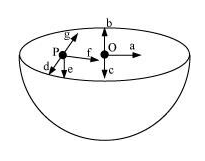Solution.(iii)
Gravitational potential ( $V$ ) is constant at all points in a spherical shell. Hence, the gravitational potential gradient $\left(\frac{d V}{d r}\right)$ is zero everywhere inside the spherical shell. The gravitational potential gradient is equal to the negative of gravitational intensity. Hence, intensity is also zero at all points inside the spherical shell. This indicates that gravitational forces acting at a point in a spherical shell are symmetric.
If the upper half of a spherical shell is cut out (as shown in the given figure), then the net gravitational force acting on a particle located at centre $\mathrm{O}$ will be in the downward direction.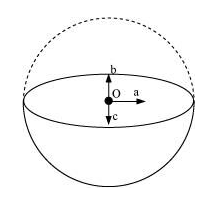Since gravitational intensity at a point is defined as the gravitational force per unit mass at that point, it will also act in the downward direction. Thus, the gravitational intensity at centre O of the given hemispherical shell has the direction as indicated by arrow c.

Question 11:
Choose the correct answer from among the given ones:
For the problem 8.10, the direction of the gravitational intensity at an arbitrary point P is indicated by the arrow (i) d, (ii) e, (iii) f, (iv) g.

Solution.
Gravitational potential $(V)$ is constant at all points in a spherical shell. Hence, the gravitational potential gradient $\left(\frac{d V}{d r}\right)$ is zero everywhere inside the spherical shell. The forces acting at a point in a spherical shell are symmetric
If the upper half of a spherical shell is cut out (as shown in the given figure), then the net gravitational force acting on a particle at an arbitrary point $\mathrm{P}$ will be in the downward direction.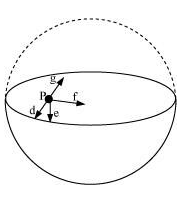Since gravitational intensity at a point is defined as the gravitational force per unit mass at that point, it will also act in the downward direction. Thus, the gravitational intensity at an arbitrary point P of the hemispherical shell has the direction as indicated by arrow e.

Question 12:
A rocket is fired from the earth towards the sun. At what distance from the earth's centre is the gravitational force on the rocket zero? Mass of the sun $=2 \times 10^{30} \mathrm{~kg}$, mass of the earth $=6 \times 10^{24} \mathrm{~kg} .$ Neglect the effect of other planets etc. (orbital radius $=1.5 \times 10^{11} \mathrm{~m}$ )

Solution
Mass of the Sun, $M_{\mathrm{s}}=2 \times 10^{30} \mathrm{~kg}$
Mass of the Earth, $M_{e}=6 \times 10^{24} \mathrm{~kg}$
Orbital radius, $r=1.5 \times 10^{11} \mathrm{~m}$
Mass of the rocket $=m$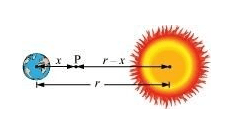Let x be the distance from the centre of the Earth where the gravitational force acting on satellite P becomes zero.
From Newton’s law of gravitation, we can equate gravitational forces acting on satellite P under the influence of the Sun and the Earth as:
$\frac{\mathrm{G} m M_{s}}{(r-x)^{2}}=\mathrm{G} m \frac{M_{e}}{x^{2}}$
$\left(\frac{r-x}{x}\right)^{2}=\frac{M_{s}}{M_{e}}$
$\frac{r-x}{x}=\left(\frac{2 \times 10^{30}}{60 \times 10^{24}}\right)^{\frac{1}{2}}=577.35$
$1.5 \times 10^{11}-x=577.35 x$
$578.35 x=1.5 \times 10^{11}$
$x=\frac{1.5 \times 10^{11}}{578.35}=2.59 \times 10^{8} \mathrm{~m}$

Question 13.
How will you 'weigh the sun', that is estimate its mass? The mean orbital radius of the earth around the sun is $1.5 \times 10^{8} \mathrm{~km}$.

Solution.
Orbital radius of the Earth around the Sun, $r=1.5 \times 10^{11} \mathrm{~m}$
Time taken by the Earth to complete one revolution around the Sun,
$T=1$ year $=365.25$ days
$=365.25 \times 24 \times 60 \times 60 \mathrm{~s}$
Universal gravitational constant, $\mathrm{G}=6.67 \times 10^{-11} \mathrm{Nm}^{2} \mathrm{~kg}^{-2}$
Thus, mass of the Sun can be calculated using the relation, \begin{aligned} M &=\frac{4 \pi^{2} r^{3}}{\mathrm{G} T^{2}} \\ &=\frac{4 \times(3.14)^{2} \times\left(1.5 \times 10^{11}\right)^{3}}{6.67 \times 10^{-11} \times(365.25 \times 24 \times 60 \times 60)^{2}} \\ &=\frac{133.24 \times 10}{6.64 \times 10^{4}}=2.0 \times 10^{30} \mathrm{~kg} \end{aligned}
Hence, the mass of the Sun is $2 \times 10^{30} \mathrm{~kg}$.

Question 14:
A Saturn year is $29.5$ times the earth year. How far is the Saturn from the sun if the earth is $1.50 \times 10^{8} \mathrm{~km}$ away from the sun?

Solution.
Distance of the Earth from the Sun, $r_{\mathrm{e}}=1.5 \times 10^{8} \mathrm{~km}=1.5 \times 10^{11} \mathrm{~m}$
Time period of the Earth $=T_{e}$
Time period of Saturn, $T_{s}=29.5 T_{e}$
Distance of Saturn from the Sun $=r_{s}$
From Kepler's third law of planetary motion, we have
$T=\left(\frac{4 \pi^{2} r^{3}}{\mathrm{G} M}\right)^{\frac{1}{2}}$
For Saturn and Sun, we can write
For Saturn and Sun, we can write
$\frac{r_{s}^{3}}{r_{e}^{3}}=\frac{T_{s}^{2}}{T_{e}^{2}}$
$r_{s}=r_{e}\left(\frac{T_{s}}{T_{e}}\right)^{\frac{2}{3}}$
$=1.5 \times 10^{11}\left(\frac{29.5 T_{e}}{T_{e}}\right)^{\frac{2}{3}}$
$=1.5 \times 10^{11}(29.5)^{\frac{2}{3}}$
$=1.5 \times 10^{11} \times 9.55$
$=14.32 \times 10^{11} \mathrm{~m}$
Hence, the distance between Saturn and the Sun is $1.43 \times 10^{12} \mathrm{~m}$.

Question 15.
A body weighs $63 \mathrm{~N}$ on the surface of the earth. What is the gravitational force on it due to the earth at a height equal to half the radius of the earth?

Solution.
Weight of the body, $W=63 \mathrm{~N}$
Acceleration due to gravity at height $h$ from the Earth's surface is given by the relation
$g^{\prime}=\frac{g}{\left(\frac{1+h}{R_{e}}\right)^{2}}$
Where,
$\mathrm{g}=$ Acceleration due to gravity on the Earth's surface
$\operatorname{Re}=$ Radius of the Earth
For $h=\frac{R_{e}}{2}$
$g^{\prime}=\frac{g}{\left(1+\frac{R_{e}}{2 \times R_{e}}\right)^{2}}=\frac{g}{\left(1+\frac{1}{2}\right)^{2}}=\frac{4}{9} g$
Weight of a body of mass $m$ at height $h$ is given as:
\begin{aligned} W^{\prime} &=m g^{\circ} \\ &=m \times \frac{4}{9} g=\frac{4}{9} \times m g \\ &=\frac{4}{9} W \\ &=\frac{4}{9} \times 63=28 \mathrm{~N} \end{aligned}

Question 16:
Assuming the earth to be a sphere of uniform mass density, how much would a body weigh half way down to the centre of the earth if it weighed 250 N on the surface?

Solution.
Weight of a body of mass $m$ at the Earth's surface, $W=m g=250 \mathrm{~N}$
Body of mass $m$ is located at depth, $d=\frac{1}{2} R_{e}$
Where,
$R_{e}=$ Radius of the Earth
Acceleration due to gravity at depth $g(d)$ is given by the relation:
$g^{\prime}=\left(1-\frac{d}{R_{e}}\right) g$
$=\left(1-\frac{R_{e}}{2 \times R_{e}}\right) g=\frac{1}{2} g$
Weight of the body at depth $d$
\begin{aligned} W^{\prime} &=m g^{\prime} \\ &=m \times \frac{1}{2} g=\frac{1}{2} m g=\frac{1}{2} W \\ &=\frac{1}{2} \times 250=125 \mathrm{~N} \end{aligned}

Question 17:
A rocket is fired vertically with a speed of $5 \mathrm{~km} \mathrm{~s}^{-1}$ from the earth's surface. How far from the earth does the rocket go before returning to the earth? Mass of the earth $=6.0 \times 10^{24}$ $\mathrm{kg}$; mean radius of the earth $=6.4 \times 10^{6} \mathrm{~m} ; \mathrm{G}=6.67 \times 10^{-11} \mathrm{~N} \mathrm{~m}^{2} \mathrm{~kg}^{-2}$

Solution.
Answer: $8 \times 10^{6} \mathrm{~m}$ from the centre of the Earth
Velocity of the rocket, $v=5 \mathrm{~km} / \mathrm{s}=5 \times 10^{3} \mathrm{~m} / \mathrm{s}$
Mass of the Earth, $M_{e}=6.0 \times 10^{24} \mathrm{~kg}$
Radius of the Earth, $R_{e}=6.4 \times 10^{6} \mathrm{~m}$
Height reached by rocket mass, $m=h$
At the surface of the Earth,
v Total energy of the rocket $=$ Kinetic energy + Potential energy
$=\frac{1}{2} m v^{2}+\left(\frac{-\mathrm{G} M_{e} m}{R_{e}}\right)$
At highest point $h$,
$v=0$
And, Potential energy $=-\frac{\mathrm{G} M_{e} m}{R_{e}+h}$
Total energy of the rocket $=0+\left(-\frac{\mathrm{G} M_{e} m}{\mathrm{R}_{e}+h}\right)=-\frac{\mathrm{G} M_{e} m}{\mathrm{R}_{e}+h}$
From the law of conservation of energy, we have
Total energy of the rocket at the Earth’s surface = Total energy at height h
$\frac{1}{2} m v^{2}+\left(-\frac{\mathrm{G} M_{e} m}{R_{e}}\right)=-\frac{\mathrm{G} M_{c} m}{R_{c}+h}$
$\frac{1}{2} v^{2}=\mathrm{G} M_{e}\left(\frac{1}{R_{e}}-\frac{1}{R_{e}+h}\right)$
$=\mathrm{G} M_{e}\left(\frac{R_{e}+h-R_{c}}{R_{e}\left(R_{e}+h\right)}\right)$
$\frac{1}{2} v^{2}=\frac{\mathrm{G} M_{e} h}{R_{e}\left(R_{c}+h\right)} \times \frac{R_{e}}{R_{e}}$
$\frac{1}{2} \times v^{2}=\frac{g R_{e} h}{R_{c}+h}$
Where $\mathrm{g}=\frac{\mathrm{G} M}{R_{e}^{2}}=9.8 \mathrm{~m} / \mathrm{s}^{2}$ (Acceleration due to gravity on the Earth's surface)
$\therefore v^{2}\left(R_{e}+h\right)=2 g R_{e} h$
$v^{2} R_{e}=h\left(2 \mathrm{~g} R_{e}-v^{2}\right)$
$h=\frac{R_{e} v^{2}}{2 g R_{e}-v^{2}}$
$=\frac{6.4 \times 10^{6} \times\left(5 \times 10^{3}\right)^{2}}{2 \times 9.8 \times 6.4 \times 10^{6}-\left(5 \times 10^{3}\right)^{2}}$v
$h=\frac{6.4 \times 25 \times 10^{12}}{100.44 \times 10^{6}}=1.6 \times 10^{6} \mathrm{~m}$
Height achieved by the rocket with respect to the centre of the Earth
$=R_{e}+h$
$=6.4 \times 10^{6}+1.6 \times 10^{6}$
$=8.0 \times 10^{6} \mathrm{~m}$

Question 18:
The escape speed of a projectile on the earth's surface is $11.2 \mathrm{~km} \mathrm{~s}^{-1}$. A body is projected out with thrice this speed. What is the speed of the body far away from the earth? lgnore the presence of the sun and other planets.

Solution.
Escape velocity of a projectile from the Earth, $v_{\mathrm{esc}}=11.2 \mathrm{~km} / \mathrm{s}$
Projection velocity of the projectile, $v_{\mathrm{p}}=3 \mathrm{v}_{\mathrm{esc}}$
Mass of the projectile $=m$
Velocity of the projectile far away from the Earth $=v_{\mathrm{f}}$
Total energy of the projectile on the Earth $=\frac{1}{2} m v_{\mathrm{p}}^{2}-\frac{1}{2} m v_{\mathrm{csc}}^{2}$
Gravitational potential energy of the projectile far away from the Earth is zero.
Total energy of the projectile far away from the Earth $=\frac{1}{2} m v_{f}^{2}$
From the law of conservation of energy, we have
$\frac{1}{2} m v_{\mathrm{p}}^{2}-\frac{1}{2} m v_{\mathrm{esc}}^{2}=\frac{1}{2} m v_{\mathrm{f}}^{2}$
$v_{\mathrm{f}}=\sqrt{v_{\mathrm{p}}^{2}-v_{\mathrm{esc}}^{2}}$
$=\sqrt{\left(3 v_{\mathrm{esc}}\right)^{2}-\left(v_{\mathrm{esc}}\right)^{2}}$
$=\sqrt{8} v_{\mathrm{esc}}$
$=\sqrt{8} \times 11.2=31.68 \mathrm{~km} / \mathrm{s}$

Question 19:
the satellite $=200 \mathrm{~kg} ;$ mass of the earth $=6.0 \times 10^{24} \mathrm{~kg}$; radius of the earth $=6.4 \times 10^{6} \mathrm{~m} ; \mathrm{G}=6.67 \times 10^{-11} \mathrm{~N} \mathrm{~m}^{2} \mathrm{~kg}^{-2}$.

Solution.
Mass of the Earth, $M=6.0 \times 10^{24} \mathrm{~kg}$
Mass of the satellite, $m=200 \mathrm{~kg}$
Radius of the Earth, $R_{\mathrm{e}}=6.4 \times 10^{6} \mathrm{~m}$
Universal gravitational constant, $\mathrm{G}=6.67 \times 10^{-11} \mathrm{Nm}^{2} \mathrm{~kg}^{-2}$
Height of the satellite, $h=400 \mathrm{~km}=4 \times 10^{5} \mathrm{~m}=0.4 \times 10^{6} \mathrm{~m}$
Total energy of the satellite at height $h=\frac{1}{2} m v^{2}+\left(\frac{-\mathrm{G} M_{e} m}{R_{e}+h}\right)$
Orbital velocity of the satellite, $v=\sqrt{\frac{\mathrm{G} M_{e}}{R_{\mathrm{e}}+h}}$'
Total energy of height, $h=\frac{1}{2} m\left(\frac{\mathrm{G} M_{\mathrm{e}}}{R_{\mathrm{e}}+h}\right)-\frac{\mathrm{G} M_{\mathrm{e}} m}{R_{\mathrm{e}}+h}=-\frac{1}{2}\left(\frac{\mathrm{G} M_{\mathrm{e}} m}{R_{\mathrm{e}}+h}\right)$
The negative sign indicates that the satellite is bound to the Earth. This is called bound energy of the satellite.
Energy required to send the satellite out of its orbit = – (Bound energy)
$=\frac{1}{2} \frac{\mathrm{G} M_{\mathrm{e}} m}{\left(R_{\mathrm{e}}+h\right)}$
$=\frac{1}{2} \times \frac{6.67 \times 10^{-11} \times 6.0 \times 10^{24} \times 200}{\left(6.4 \times 10^{6}+0.4 \times 10^{6}\right)}$
$=\frac{1}{2} \times \frac{6.67 \times 6 \times 2 \times 10}{6.8 \times 10^{6}}=5.9 \times 10^{9} \mathrm{~J}$

Question 20:
Two stars each of one solar mass $\left(=2 \times 10^{30} \mathrm{~kg}\right)$ are approaching each other for a head on collision. When they are a distance $109 \mathrm{~km}$, their speeds are negligible. What is the speed with which they collide? The radius of each star is $104 \mathrm{~km}$. Assume the stars to remain undistorted until they collide. (Use the known value of $\mathrm{G}$ )
Solution.
Mass of each star, $M=2 \times 10^{30} \mathrm{~kg}$
Radius of each star, $R=10^{4} \mathrm{~km}=10^{7} \mathrm{~m}$
Distance between the stars, $r=10^{9} \mathrm{~km}=10^{12} \mathrm{~m}$
For negligible speeds, $v=0$ total energy of two stars separated at distance $r$
$=\frac{-\mathrm{G} M M}{r}+\frac{1}{2} m v^{2}$
$=\frac{-\mathrm{G} M M}{r}+0 \quad \cdots$(1)
Now, consider the case when the stars are about to collide:
Velocity of the stars $=v$
Distance between the centers of the stars $=2 R$
Total kinetic energy of both stars $=\frac{1}{2} M v^{2}+\frac{1}{2} M v^{2}=M v^{2}$
Total potential energy of both stars $=\frac{-\mathrm{G} M M}{2 R}$
\text { Total energy of the two stars }=M v^{2}-\frac{G M M}{2 R}... (ii)
Using the law of conservation of energy, we can write:
$M v^{2}-\frac{\mathrm{G} M M}{2 R}=\frac{-\mathrm{G} M M}{r}$
$v^{2}=\frac{-\mathrm{G} M}{r}+\frac{\mathrm{G} M}{2 R}=\mathrm{G} M\left(-\frac{1}{r}+\frac{1}{2 R}\right)$
$=6.67 \times 10^{-11} \times 2 \times 10^{30}\left[-\frac{1}{10^{12}}+\frac{1}{2 \times 10^{7}}\right]$
$$=13.34 \times 10^{19}\left[-10^{-12}+5 \times 10^{-8}\right]$$
$\sim 13.34 \times 10^{19} \times 5 \times 10^{-8}$
$\sim 6.67 \times 10^{12}$
$v=\sqrt{6.67 \times 10^{12}}=2.58 \times 10^{6} \mathrm{~m} / \mathrm{s}$

Question 21:
Two heavy spheres each of mass 100 kg and radius 0.10 m are placed 1.0 m apart on a horizontal table. What is the gravitational force and potential at the mid point of the line joining the centers of the spheres? Is an object placed at that point in equilibrium? If so, is the equilibrium stable or unstable?

Solution.
$0 ;$
$-2.7 \times 10^{-8} \mathrm{~J} / \mathrm{kg}$
Yes;
Unstable
Explanation:
The situation is represented in the given figure: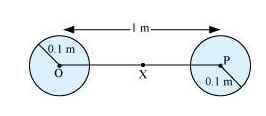Mass of each sphere, M = 100 kg
Separation between the spheres, r = 1m
X is the mid point between the spheres. Gravitational force at point X will be zero. This is because gravitational force exerted by each sphere will act in opposite directions.
Gravitational potential at point X:
$=\frac{-\mathrm{G} M}{\left(\frac{r}{2}\right)}-\frac{\mathrm{G} M}{\left(\frac{r}{2}\right)}=-4 \frac{\mathrm{G} M}{r}$
$=\frac{4 \times 6.67 \times 10^{-11} \times 100}{1}$
$=-2.67 \times 10^{-8} \mathrm{~J} / \mathrm{kg}$
Any object placed at point X will be in equilibrium state, but the equilibrium is unstable. This is because any change in the position of the object will change the effective force in that direction.

Question 22:
As you have learnt in the text, a geostationary satellite orbits the earth at a height of nearly $36,000 \mathrm{~km}$ from the surface of the earth. What is the potential due to earth's gravity at the site of this satellite? (Take the potential energy at infinity to be zero). Mass of the earth $=6.0 \times 10^{24} \mathrm{~kg}$, radius $=6400 \mathrm{~km}$

Solution.
Mass of the Earth, $M=6.0 \times 10^{24} \mathrm{~kg}$
Radius of the Earth, $R=6400 \mathrm{~km}=6.4 \times 10^{6} \mathrm{~m}$
Height of a geostationary satellite from the surface of the Earth,
$h=36000 \mathrm{~km}=3.6 \times 10^{7} \mathrm{~m}$
Gravitational potential energy due to Earth's gravity at height $h_{,}$
$=\frac{-\mathrm{G} M}{(R+h)}$
$=-\frac{6.67 \times 10^{-11} \times 6.0 \times 10^{24}}{3.6 \times 10^{7}+0.64 \times 10^{7}}$
$=-\frac{6.67 \times 6}{4.24} \times 10^{13-7}$
$=-9.4 \times 10^{6} \mathrm{~J} / \mathrm{kg}$

Question 23:
A star 2.5 times the mass of the sun and collapsed to a size of 12 km rotates with a speed of 1.2 rev. per second. (Extremely compact stars of this kind are known as neutron stars.
Certain stellar objects called pulsars belong to this category). Will an object placed on its equator remain stuck to its surface due to gravity? (Mass of the sun $\left.=2 \times 10^{30} \mathrm{~kg}\right)$.

Solution.
A body gets stuck to the surface of a star if the inward gravitational force is greater than the outward centrifugal force caused by the rotation of the star.
Gravitational force, $f_{\mathrm{g}}=\frac{\mathrm{G} M m}{R^{2}}$
Where,
$M=$ Mass of the star $=2.5 \times 2 \times 10^{30}=5 \times 10^{30} \mathrm{~kg}$
$m=$ Mass of the body
$R=$ Radius of the star $=12 \mathrm{~km}=1.2 \times 10^{4} \mathrm{~m}$
$\therefore f_{\mathbb{g}}=\frac{6.67 \times 10^{-11} \times 5 \times 10^{30} \times m}{\left(1.2 \times 10^{4}\right)^{2}}=2.31 \times 10^{11} \mathrm{~m} \mathrm{~N}$
Centrifugal force, $f_{\mathrm{c}}=m r \omega^{2}$
$\omega=$ Angular speed $=2 \pi v$
$\mathrm{v}=$ Angular frequency $=1.2 \mathrm{rev} \mathrm{s}^{-1}$
$f_{\mathrm{C}}=m R(2 \pi v)^{2}$
$=m \times\left(1.2 \times 10^{4}\right) \times 4 \times(3.14)^{2} \times(1.2)^{2}=1.7 \times 10^{5} \mathrm{mN}$
Since $f_{\mathrm{g}}>f_{\mathrm{c}}$, the body will remain stuck to the surface of the star.

Question 24:
A spaceship is stationed on Mars. How much energy must be expended on the spaceship to launch it out of the solar system? Mass of the space ship = $1000 \mathrm{~kg}$; mass of the Sun $=2 \times 10^{30} \mathrm{~kg}$; mass of mars $=6.4 \times 10^{23} \mathrm{~kg}$; radius of mars $=3395 \mathrm{~km}$; radius of the orbit of mars $=2.28 \times 10^{8} \mathrm{~kg} ; \mathrm{G}=6.67 \times 10^{-11} \mathrm{~m}^{2} \mathrm{~kg}^{-2}$

Solution.
Mass of the spaceship, $m_{\mathrm{s}}=1000 \mathrm{~kg}$
Mass of the Sun, $M=2 \times 10^{30} \mathrm{~kg}$
Mass of Mars, $m_{m}=6.4 \times 10^{23} \mathrm{~kg}$
Orbital radius of Mars, $R=2.28 \times 10^{8} \mathrm{~kg}=2.28 \times 10^{11} \mathrm{~m}$
Radius of Mars, $r=3395 \mathrm{~km}=3.395 \times 10^{6} \mathrm{~m}$
Universal gravitational constant, $G=6.67 \times 10^{-11} \mathrm{~m}^{2} \mathrm{~kg}^{-2}$
Potential energy of the spaceship due to the gravitational attraction of the Sun $=\frac{-\mathrm{G} M m_{\mathrm{s}}}{R}$
Potential energy of the spaceship due to the gravitational attraction of Mars $=\frac{-\mathrm{G} M_{m} m_{\mathrm{s}}}{r}$
Since the spaceship is stationed on Mars, its velocity and hence, its kinetic energy will be zero.
Total energy of the spaceship $=\frac{-\mathrm{G} M m_{\mathrm{s}}}{R}-\frac{-\mathrm{G} M_{\mathrm{s}} m_{\mathrm{m}}}{r}$
$=-\mathrm{G} m_{\mathrm{s}}\left(\frac{M}{R}+\frac{m_{\mathrm{m}}}{r}\right)$
Energy required for launching the spaceship out of the solar system
$=-$ (Total energy of the spaceship)
$=\mathrm{G} m_{\mathrm{s}}\left(\frac{M}{R}+\frac{m_{m}}{r}\right)$
$=6.67 \times 10^{-11} \times 10^{3} \times\left(\frac{2 \times 10^{30}}{2.28 \times 10^{11}}+\frac{6.4 \times 10^{23}}{3.395 \times 10^{6}}\right)$
$=6.67 \times 10^{-8}\left(87.72 \times 10^{17}+1.88 \times 10^{17}\right)$
$=6.67 \times 10^{-8} \times 89.50 \times 10^{17}$
$=596.97 \times 10^{9}$
$=6 \times 10^{11} \mathrm{~J}$

Question 25:
A rocket is fired 'vertically' from the surface of mars with a speed of $2 \mathrm{~km}$ s-1. If $20 \%$ of its initial energy is lost due to Martian atmospheric resistance, how far will the rocket go from the surface of mars before returning to it? Mass of mars $=6.4 \times 1023 \mathrm{~kg}$; radius of mars $=3395 \mathrm{~km} ; \mathrm{G}=6.67 \times 10^{-11} \mathrm{~N} \mathrm{~m}^{2} \mathrm{~kg}^{-2}$

Solution.
Initial velocity of the rocket, $v=2 \mathrm{~km} / \mathrm{s}=2 \times 10^{3} \mathrm{~m} / \mathrm{s}$
Mass of Mars, $M=6.4 \times 10^{23} \mathrm{~kg}$
Radius of Mars, $R=3395 \mathrm{~km}=3.395 \times 10^{6} \mathrm{~m}$
Universal gravitational constant, $G=6.67 \times 10^{-11} \mathrm{~N} \mathrm{~m}^{2} \mathrm{~kg}^{-2}$
Mass of the rocket $=m$
Initial kinetic energy of the rocket $=\frac{1}{2} m v^{2}$
Initial potential energy of the rocket $=\frac{-\mathrm{G} M m}{R}$
Total initial energy $=\frac{1}{2} m v^{2}-\frac{\mathrm{G} M m}{R}$
If $20 \%$ of initial kinetic energy is lost due to Martian atmospheric resistance, then only $80 \%$ of its kinetic energy helps in reaching a height.
Total initial energy available $=\frac{80}{100} \times \frac{1}{2} m v^{2}-\frac{\mathrm{G} M m}{R}=0.4 m v^{2}-\frac{\mathrm{G} M m}{R}$
Maximum height reached by the rocket $=h$
At this height, the velocity and hence, the kinetic energy of the rocket will become zero.
Total energy of the rocket at height $h=-\frac{\mathrm{G} M m}{(R+h)}$
Applying the law of conservation of energy for the rocket, we can write:
$0.4 m v^{2}-\frac{\mathrm{GMm}}{R}=\frac{-\mathrm{G} M m}{(R+h)}$
$0.4 v^{2}=\frac{\mathrm{G} M}{R}-\frac{\mathrm{G} M}{R+h}$
$=\mathrm{G} M\left(\frac{1}{R}-\frac{1}{R+h}\right)$
$=\mathrm{G} M\left(\frac{R+h-R}{R(R+h)}\right)$
$=\frac{\mathrm{G} M h}{R(R+h)}$
$\frac{R+h}{h}=\frac{\mathrm{G} M}{0.4 v^{2} R}$
$\frac{R}{h}+1=\frac{\mathrm{G} M}{0.4 v^{2} R}$
$\frac{R}{h}=\frac{\mathrm{G} M}{0.4 v^{2} R}-1$
$h=\frac{R}{\frac{\mathrm{G} M}{0.4 v^{2} R}-1}$
$=\frac{0.4 R^{2} v^{2}}{\mathrm{GM}-0.4 v^{2} R}$
$=\frac{0.4 \times\left(3.395 \times 10^{6}\right)^{2} \times\left(2 \times 10^{3}\right)^{2}}{6.67 \times 10^{-11} \times 6.4 \times 10^{23}-0.4 \times\left(2 \times 10^{3}\right)^{2} \times\left(3.395 \times 10^{6}\right)}$
$=\frac{18.442 \times 10^{18}}{42.688 \times 10^{12}-5.432 \times 10^{12}}=\frac{18.442}{37.256} \times 10^{6}$
$=495 \times 10^{3} \mathrm{~m}=495 \mathrm{~km}$